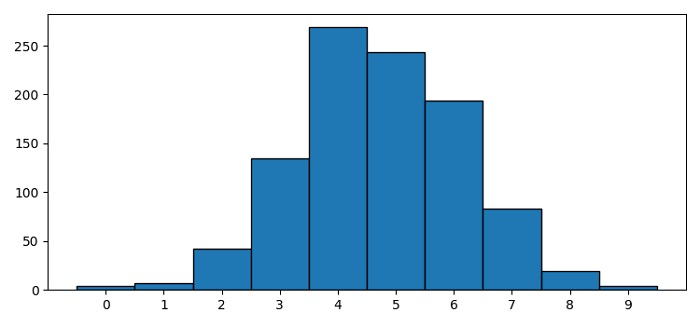# How to center labels in a Matplotlib histogram plot?

To place the labels at the center in a histogram plot, we can calculate the mid-point of each patch and place the ticklabels accordinly using xticks() method.

## Steps

• Set the figure size and adjust the padding between and around the subplots.

• Create a random standard sample data, x.

• Initialize a variable for number of bins.

• Use hist() method to make a histogram plot.

• Calculate the list of ticks at the center of each patch.

• Make a list of tickslabels.

• Use xticks() method to place xticks and labels.

• To display the figure, use show() method.

## Example

import numpy as np
from matplotlib import pyplot as plt
plt.rcParams["figure.figsize"] = [7.50, 3.50]
plt.rcParams["figure.autolayout"] = True
x = np.random.randn(1000)
n_bins = 10
n, bins, patches = plt.hist(x, bins=n_bins, edgecolor='black')
ticks = [(patch._x0 + patch._x1)/2 for patch in patches]
ticklabels = [i for i in range(n_bins)]
plt.xticks(ticks, ticklabels)
plt.show()

## Output